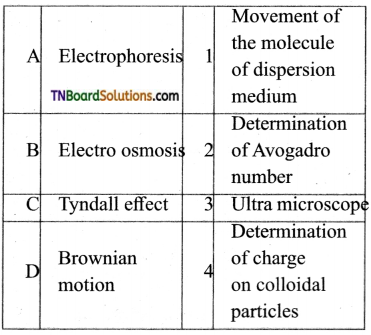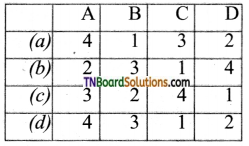Students get through the TN Board 12th Chemistry Important Questions Chapter 10 Surface Chemistry which is useful for their exam preparation.

## TN State Board 12th Chemistry Important Questions Chapter 10 Surface Chemistry

Question 1.

3. Charcoal adsorbs colorants from sugar.

Question 2.
Adsorption is a surface phenomenon while absorption is a bulk phenomenon, i.e., the adsorbate molecules are distributed throughout the adsorbent.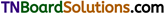Question 3.
Examples for adsorbates: The gaseous molecules like He, Ne, O2, N2, SO2 and NH3 and solutions of NaCl or KCl.
Examples for adsorbents: Silica gel, metals like Ni, Cu, Pt, Ag and Pd and certain colloids.

Question 4.
What is an interface?
The surface of separation of two phases where the concentration of adsorbed molecules is high is known as interface.

Question 5.

1. Adsorption can occur in all interfacial surfaces i.e., the adsorption can occur in between gas-solid, liquid solid, liquid- liquid, solid- solid and gas-liquid.
2. Adsorption is always accompanied by decrease in free energy. When ΔG reaches zero, the equilibrium is attained.
3. Adsorption is a spontaneous process.
4. When molecules are adsorbed, there is always a decrease in randomness of the molecules.
We know, ΔG = ΔH – T ΔS where ΔG is change in free energy.
ΔH is change in enthalpy and ΔS is change in entropy.
Hence, ΔH = ΔG + TΔS Adsorption is exothermic as there is an interaction between adsorbate and adsorbent.Question 6.
What do you understand by the term ‘sorption’?
The term represents simultaneous adsorption and absorption.

Question 7.
What is the term used for sorption of gases on metal surfaces?
Occlusion.

Question 8.
Give three examples for chemical adsorption or chemisorption.

1. Adsorption of O2 on tungsten.
2. Adsorption of H2 on nickel.
3. Adsorption of ethyl alcohol vapours on nickel.

Question 9.
Give two examples for physical adsorption.

1. Adsorption of N2 on mica.
2. Adsorption of gases on charcol.

Question 10.
Explain the nature of the force of attraction that exists between an adsorbent and an adsorbate in (i) physical adsorption and (ii) chemical adsorption.
(i) Physical adsorption are (a) vander waals force of attraction, (b) dipole-dipole interaction and (c) dispersion forces.
(ii) In chemisorption gas molecules are held to the surface by formation of chemical bonds. Since strong bonds are formed, nearly 400 kJ mole is given out as heat of adsorption.Question 11.
When adsorption occurs, entropy decreases, i.e., ΔS = negative. As ΔG = ΔH – TΔS, for a spontaneous process, AG must be negative. This can only be so, if ΔH is negative, i.e., the process is exothermic.

Question 12.
How do size of particles of adsorbent, pressure of gas and prevailing temperature influence the extent of adsorption of a gas on a solid?

1. The smaller the size of the particles of the adsorbent, greater is the surface area, greater is the adsorption.
2. At constant temperature, adsorption first increases with increase in pressure and then attains equilibrium at high pressures.
3. In physical adsorption, it decreases with increase in temperatures, but in chemisorption first, it increases and then decreases.

Question 13.
What are adsorption isotherms and isobars? Explain.
The plot of the amount of adsorption verses temperature at constant pressure is called adsorption isobar. The adsorption isobars of physisorption and chemisorption as shown in figure.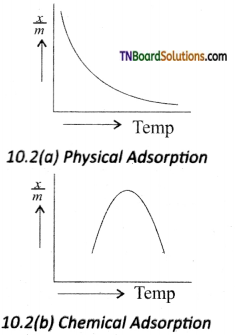In physical adsorption, $$\frac{x}{m}$$ decreases with increases in T, But in chemical adsorption, $$\frac{x}{m}$$ increases with rise in temperature and then decreases. The increase illustrates the requirement of activation of the surface for adsorption is due to fact that the formation of activated complex requires certain energy.
The decrease at high temperature is due to desorption, as the kinetic energy of the adsorbate increases.Question 14.
In order to explain these isotherms, various equations were suggested as follows: Freundlich adsorption isotherm.
According to Freundlinch,
$$\frac{x}{m}$$ = Kp$$\frac{1}{n}$$
where V is the amount of adsorbate or adsorbed on ‘m’ gm of adsorbent at a pressure of p. K and n are constants.
Value n is always less than unity.
This equation is applicable for adsoiption of gases on solid surfaces. The same equation becomes $$\frac{x}{m}$$ = Kc$$\frac{1}{n}$$ when used for adsorption in solutions with c as concentration.
This equation quantitively predict the effect of pressure (or concentration) on the adsorption of gases (or adsorbates) at a constant temperature.
Taking log on both sides of the equation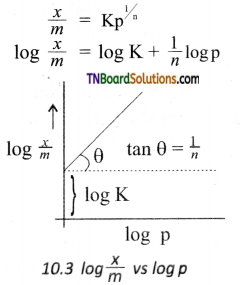Hence the intercept represents the value of log K and the slope $$\frac{b}{q}$$ gives $$\frac{1}{n}$$.
This equation explains the increase of $$\frac{x}{m}$$ with an increase in pressure. But experimental values show the deviation at low pressure.

Question 15.
Explain the application of adsorption (i) in the use of gas masks, (ii) in the use of softening hard water, (iii) in decolourisation of sugar.
(i) Gas masks are devices containing suitable adsorbents so that poisonous gases present in the atmosphere are preferentially adsorbed and the air for breathing is purified.
Activated charcoal is one of the best adsorbents.
(ii) Permutit is employed for this process which adsorbs Ca2+ and Mg2+ ions in its surface, there is an ion exchange as shown below it occurs on the surface.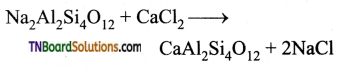Exhausted permutit is regenerated by adding a solution of common salt.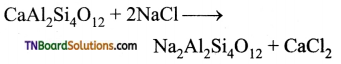(iii) Sugar prepared from molasses is decolourised to remove coloured impurities by adding animal charcoal which acts as decolourising material.Question 16.
In the precipitation titrations, the endpoint is indicated by an external indicator that changes its colour after getting adsorbed on precipitate. It is used to indicate the endpoint of the titration.

Question 17.
Distinguish between homogeneous catalysis and heterogeneous catalysis with suitable examples.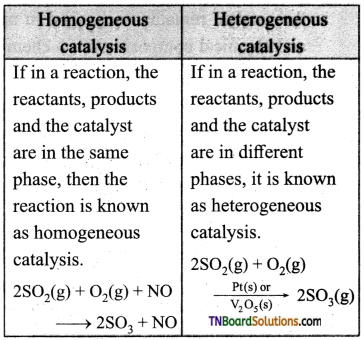Question 18.
Name the catalysts used in the following:
(i) Haber’s process in the manufacture of ammonia.
(ii) Oxidation of ammonia.
(iii) Hydrogenation of alkenes.
(iv) Decomposition of H2O2.
(v) Formation of acetophenone from benzene and ethanoyl chloride. Also, give equations for the reactions.
(i) The catalyst used is Iron,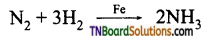(ii) The catalyst is platinum gauze.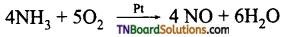(iii) The catalyst is finely divided nickel.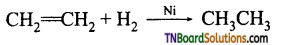(iv) The catalyst is platinum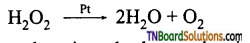(v) The catalyst is anhydrous aluminium chloride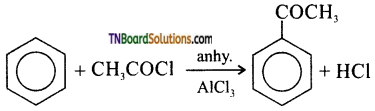Question 19.
Mention the characteristics of catalysts.

1. For a chemical reaction, the catalyst is needed in very small quantities. Generally, a pinch of catalyst is enough for a reaction in bulk.
2. There may be some physical changes, but the catalyst remains unchanged in mass and chemical composition in a chemical reaction.
3. A catalyst itself can not initiate a reaction. It means it can not start a reaction that is not taking place. But, if the reaction is taking place at a slow rate it can increase its rate.
4. A solid catalyst will be more effective if it is taken in a finely divided form.
5. A catalyst can catalyse a particular type of reaction, hence they are said to be specific in nature.
6. In an equilibrium reaction, the presence of catalyst reduces the time for attainment of equilibrium and here it does not affect the position of equilibrium and the value of the equilibrium constant.
7. A catalyst is highly effective at a particular temperature called as optimum temperature.
8. The presence of a catalyst generally does not change the nature of products
eg: 2SO2 + O2 → 2SO3
This reaction is slow in the absence of a catalyst, but fast in the presence if Pt catalyst.Question 20.
What are promoters? Explain with an example.
In a catalysed reaction the presence of a certain substance increases the activity of a catalyst. Such a substance is called a promoter. For example in the Haber’s process of manufacture ammonia, the activity of the iron catalyst is increased by the presence of molybdenum. Hence molybdenum is called a promoter. In the same way, Al2O3 can also be used as a promoter to increase the activity of the iron catalyst.

Question 21.
Explain the action of anhydrous aluminium chloride in Friedel-Crafts reaction.
The mechanism of Friedel crafts reaction is given below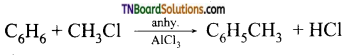The action of catalyst is explained as follows: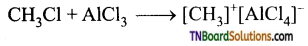It is an intermediate.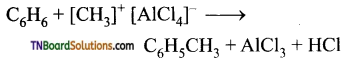Question 22.
Give the mechanism of the following reactions based on intermediate formation theory.

1. Thermal decomposition of KClO3 in the presence of MnO2.
Steps in the reaction
2KClO3 → 2KCl + 3O2
can be given as
2KClO3 + 6MnO2 → 6MnO3 + 2KCl
It is an intermediate,
6MnO3 → 6MnO2 + 3O2
2. The reaction between H2 and O2 in the presence of copper.
2Cu + $$\frac{1}{2}$$ O2 → Cu2O It is an intermediate.
Cu2O + H2 → H2O + 2Cu
3. Oxidation of HCl by air in the presence of CuCl2.
2CuCl2 → Cl2 + Cu2Cl2
2Cu2Cl2 + O2 → 2Cu2OCl2
It is an intermediate.
2Cu2OCl2 + 4HCl → 2H2O + 4CuCl2
This theory describes
(a) the specificity of a catalyst and
(b) the increase in the rate of the reaction with an increase in the concentration of a catalyst.Question 23.
Mention the limitations of the intermediate compound formation theory of catalysis.

1. The intermediate compound theory fails to explain the action of catalytic poison and activators (promotors).
2. This theory is unable to explain the mechanism of heterogeneous catalysed reactions.

Question 24.
Explain the salient features of the adsorption theory of catalysis.
According to this theory, the reactants are adsorbed on the catalyst surface to form an activated complex which subsequently decomposes and gives the product.
The various steps involved in a heterogeneous catalysed reaction are given as follows:

1. Reactant molecules diffuse from bulk to the catalyst surface.
2. The reactant molecules are adsorbed on the surface of the catalyst.
3. The adsorbed reactant molecules are activated and form an activated complex which is decomposed to form the products.
4. The product molecules are desorbed.
5. The product diffuses away from the surface of the catalyst.

Question 25.
What are active centres? How does the presence of active centres influence the rate of catalysed reaction?
The surface of a catalyst is not smooth. It bears steps, cracks and comers. Hence the atoms on such locations of the surface are coordinatively unsaturated. So, they have much residual force of attraction. Such sites are called active centres. So, the surface carries high surface free energy.
The presence of such active centres increases the rate of reaction by adsorbing and activating the reactants.
The adsorption theory explains the following

1. Increase in the activity of a catalyst by increasing the surface area. An increase in the surface area of metals and metal oxides by reducing the particle size increases the rate of the reaction.
2. The action of catalytic poison occurs when the poison blocks the active centres of the catalyst.
3. A promoter or activator increases the number of active centres on the surfaces.

Question 26.
What is the role of a promoter in heterogeneous catalysis?
A promoter increases the number of active centres on the surfaces.

Question 27.
What are catalytic poisons? How do they affect a catalysed reaction?
Substances that destroy the activity of catalysts are known as catalytic poisons. They affect the catalytic reaction by occupying the active centres of the catalyst as a result active centres will not available for adsorption by reactants.

Question 28.
Give some examples of enzyme catalyses. Some common examples for enzyme catalysis.

1. The peptide glycyl L-glutamyl L-lyrosin is hydrolysed by an enzyme called pepsin.
2. The enzyme diastase hydrolyses starch into maltose.
2(C6H10O5)n + nH2O → nC2H22O11
3. The yeast contains the enzyme zymase which converts glucose into ethanol.
C6H12O6 + H2O → 2C2H5OH + 2CO2
4. The enzyme micoderma aceti oxidises alcohol into acetic acid.
C2H5OH + O2 → CH3COOH + H2O
5. The enzyme urease present in soya beens hydrolyses the urea.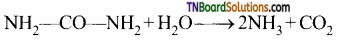Question 29.
Briefly outline the characteristics of enzyme catalysed reaction.

1. An enzyme catalyst is specific in nature, i.e., one catalyst cannot catalyse more than one reaction.
2. The enzyme catalysed reactions are very fast compared to other catalysed reactions.
3. The rate of an enzyme-catalysed reaction depends on temperature. At an optimum temperature, the rate of the reaction becomes maximum. Beyond the optimum temperate, the rate decrease.
4. The enzyme activity is maximum at a particular pH called optimum pH. The favourable pH range for enzymatic reactions is 5-7.
5. The enzymatic activity is increased by the presence of containing substances, known as coenzymes.
6. The activity of enzymes can also be inhibited or poisoned due to the presence of certain substances.

Question 30.
Explain phase transfer catalysis with an example.
Substitution of Cl and CN in the following reaction.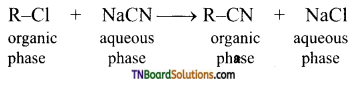R-Cl = 1-chlorooctane
R-CN = 1-cyanooctane
By direct heating of two-phase mixture of organic 1 -chlorooctane with aqueous sodium cyanide for several days, 1-cyanooctane is not obtained. However, if a small amount of quaternary ammonium salt like tetraalkyl ammonium chloride is added, a rapid transition of 1 -cyanooctane occurs in about 100% yield after 1 or 2 hours. In this reaction, the tetraalkyl ammonium cation, which has hydrophobic and hydrophilic ends, transports CN from the aqueous phase to the organic phase using its hydrophilic end and facilitates the reaction with 1-chloroocatne as shown below: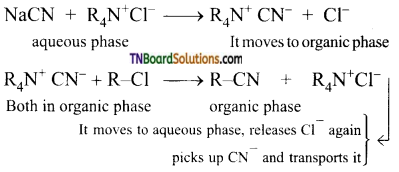So phase transfer catalyst speeds up the reaction by transporting one reactant from one phase to another.

Question 31.
Name the dispersed phase and dispersed medium in the following: fog, dust, sharing cream, milk, paints.

 Example Dispersed phase Dispersion medium Fog Liquid Gas Dust Solid Gas Sharing cream Gas Liquid Milk Liquid Liquid Paint Solid Liquid

Question 32.
Give the name of the colloid and one example for (i) a gas dispersed in solid, (ii) a liquid dispersed in a solid, (iii) a solid dispersed in a solid.
(i) A gas dispersed in a solid is known as a solid foam. Pumice stone, rubber, bread are examples.
(ii) A liquid dispersed in a solid is known as a gel. Butter and cheese are examples.
(iii) A solid dispersed in another solid is known as a solid sol. Pearls, coloured glass, alloys are examples of solid sols.Question 33.
Briefly outline the principle involved in the preparation of colloidal solution by dispersion methods.
In the dispersion method, the particles whose size is higher than that of colloidal particles are disintegrated to that of the colloidal particles subjecting it to a mechanical disintegration in a colloid mill or by electro-dispersion.

Question 34.
Explain how colloidal particles of metals are prepared by electro dispersion method.
An electrical arc is struck between electrodes dispersed in the water surrounded by ice. When a current of 1 amp /100V is passed an arc produced forms vapours of metal which immediately condense to form a colloidal solution. By this method colloidal solution of many metals like copper, silver, gold, platinum, etc. can be prepared Alkali hydroxide is added as a stabilising agent for the colloidal solution.

Question 35.
Explain peptisation with an example.
The conversion of a freshly precipitated substance into a colloid by the addition of an electrolyte (peptising agent) is known as peptisation. For example, freshly precipitated silver chloride is converted into a colloidal solution by the addition of HCl.

Question 36.
Explain the principle involved in the preparation of colloids by the condensation method.
Particles whose size are lesser than the colloidal particles are brought together to the size of colloidal particles.

Question 37.
What is the principle involved in the purification of a colloidal solution by dialysis?
The separation of crystalloids (electrolytes) from colloids is based upon the principle that particles of crystalloid pass through the animal membrane (bladder) or parchment paper, or cellophane sheet whereas those of colloid do not.Question 38.
Explain why? (i) colloidal particles are not visible to the naked eye, (ii) colloidal particles pass through ordinary filter paper, (iii) the sky appears blue, (iv) finest gold sol is red in colour.
(i) No particle is visible to the naked eye if its diameter is less than half the wavelength of light used. The shortest wavelength of light is about 4000Å or 400 mμ. Hence no particle of diameter less than 200 mμ can be seen. The size of the colloidal particles is less than 200 mμ.
(ii) The size of the colloidal particles are smaller than the pores in the filter paper and hence, they pass through the filter paper.
(iii) This is due to the Tyndall effect. The colloidal particles absorb light energy and then scatter in all directions.
(iv) The colour of the colloidal solution depends as the wavelength of scattered radiation by dispersed particles. The wavelength of light further depends on the size and nature of particles. The finest gold sol is red in colour. As the size of particles increase, it becomes purple, then blue and finally golden yellow.

Question 39.
Explain the Tyndall effect.
Colloids have optical properties. When a homogeneous solution is seen in the direction of light, it appears clear but it appears dark, in a perpendicular direction.
But when light passes through a colloidal solution, it is scattered in all directions. The colloidal particles absorb a portion of light and the remaining portion is scattered from the surface of the colloid. Hence the path of light is made clear. This phenomenon is known as the Tyndall effect.

Question 40.
Give an account of the Brownian movement.
The zig-zag random motion of colloidal particles, when viewed through ultramicroscope, is known as Brownian movement. The reason for Brownian movement is that the colloidal sol particles are continuously bombarded with the molecules of the dispersion medium and hence they follow a zigzag, random, continuous movement.
Brownian movement enables us,
II. to confirm kinetic theory which considers the ceaseless rapid movement of molecules that increases with an increase in temperature.
III. to understand the stability of colloids: As the particles are in continuous rapid movement they do not come close and hence not get condensed. That is Brownian movement does not allow the particles to be acted on by force of gravity.

Question 41.
Explain the term electrophorosis.
The movement of colloidal particles towards cathode or anode depending on the charge of the particles under the influence of electric current is known as electrophorosis.
This experiment is used to detect the charge on the colloidal particles. If the colloidal particles move towards the cathode, it is negatively charged. If they are positively charged, they migrate towards anode.Question 42.
Give examples for positively charged and negatively charged colloids.

 Positively charge colloids Negatively charge colloids Ferric hydroxide Ag, Au & Pt Aluminium hydroxide Arsenic sulphide Basic dyes Clay Haemoglobin Starch

Question 43.
Account for the charge on colloidal particles.
The colloidal particles, in whichever method is prepared, contain traces of electrolytes. It preferentially adsorbs either positive or negative ions from the solution. If the particles adsorb positive ions from the solution, it becomes a positively charged colloid. If it adsorbs a negative ion from the solution, it becomes a negatively charged colloid.

Question 44.
Account for the stability of a colloid.
Since the colloidal particles are either positive or negatively charged, they do not come close together. They repel each other. Thus, the charge on the colloidal particles is responsible for its stability.

Question 45.
Explain the term coagulation or flocculation.
The conversion of a colloid to a precipitate is known as coagulation or precipitation. Coagulation results in the removal of charges on the colloid by any one of the following:

2. electrophoresis
3. mixing oppositely charged sols
4. boiling.

These methods help remove charges from the particles. Thus, the particles come closer, form an aggregate of particles and finally settles down as a precipitate due to gravity.

Question 46.
The peptising agent is added to convert a precipitate into a colloidal solution. Give reason.
Ions (either positive or negative) of the peptising agent (electrolyte) are adsorbed on the particles of the precipitate. They repel and hit each other breaking the particles of the precipitate into colloidal size.

Question 47.
Explain what is observed (i) when a beam of light is passed through a colloidal solution.
(ii) an electrolyte, NaCl is added to ferric hydroxide solution,
(iii) electric current is passed through a colloidal solution.
(i) Scattering of light by colloidal particles take place and the path of light becomes visible.
(ii) The positively charged colloidal particles of Fe(OH)3 gets coagulated by the oppositely charged Cl ions provided by NaCl.
(iii) On passing electric current, colloidal particles move towards the oppositely charged electrode where they lose their charge Cl get coagulated.Question 48.
What happens when gelatin is mixed with gold sol?
Gold sol is lyophobic. Gelatin forms lyophilic sol and acts as a protective colloid. On adding gelatin to gold sol, the latter becomes more stable.

Question 49.
A colloid is formed by adding FeCl3 in excess of hot water. What will happen if an excess ferric chloride is added to this colloid?
On adding FeCl3 to water, a colloidal sol of hydrated ferric oxide is formed. This is a positively charged colloid as it adsorbs Fe+3 ions on its surface. On adding NaCl, Cl ions bring about coagulation.

Question 50.
How does the boiling of a colloid, help the coagulation of a colloidal solution?
Boiling vessels in increased collisions between the colloidal particles. The sol particles combine and settle down as a precipitate.

Question 51.
Explain the protective action of lyophilic colloids.
The addition of a lyophobic colloid into lyophilic colloid protects the latter from coagulation. Hence, lyophilic colloids act as protective colloids.

Question 52.
Define gold number. Mention its use.
‘Gold number’ as a measure of protecting the power of a colloid. The gold number is defined as the number of milligrams of hydrophilic colloid that will just prevent the precipitation of 10ml of gold sol on the addition of 1ml of 10% NaCl solution. Smaller the gold number greater the protective power.

Question 53.
What are emulsions? Mention various types of emulsions.
Emulsions are a colloidal solutions in which a liquid is dispersed in another liquid.
Generally, there are two types of emulsions.

1. Oil in Water (O/W)
2. Water in Oil (W/O)Question 54.
Give one example each for (i) oil in water and (ii) water in oil emulsions.
(i) In Oil in Water (O/W) emulsions, the dispersed phase is oil and the dispersion medium is water.
eg: Milk is an emulsion of liquid fat dispersed in water and vanishing cream.
(ii) Emulsion of Water in Oil (W/O) in which water is the dispersed phase and oil is the dispersion medium.
eg: Cod liver oil, butter and cold cream.

Question 55.
What is emulsification?
The process of preparation of emulsion by the dispersal of one liquid in another liquid is called Emulsification.

Question 56.
What is an emulsifier or emulsification agent?
It is a substance added to have a stable emulsion. The type of emulsion formed depends upon the nature of the emulsifying agent. For example, the presence of soluble soaps as an emulsifying agent favours the formation of oil in water type of emulsions, whereas insoluble soaps (containing non alkali metal atoms) favours the formation of water in oil emulsions.

Question 57.
Mention the properties of the emulsion.

1. Emulsions exhibit all the properties like the Tyndall effect, Brownian movement, Electrophoresis, Coagulation on the addition of electrolytes.
2. Emulsions can be separated into their constituent liquids by boiling, freezing, centrifuging, electrostatic precipitation by adding large amounts of the electrolytes to precipitate out the dispersed phase or by chemical destruction of emulsifying agent.
3. Emulsions can be diluted by adding any amount of dispersion medium.

1. Which of the following is true with respect of adsorption?
(a) ΔG < 0, ΔS > 0, ΔH < 0
(b) ΔG < 0, ΔS < 0, ΔH < 0
(c) ΔG > 0, ΔS > 0, ΔH < 0
(d) ΔG < 0, ΔS < 0, ΔH > 0
(b)2. Which of the following statements is incorrect regarding physisorption?
(a) It occurs because of vander Waals forces.
(c) Under high pressure, it results in multimolecular layer on the adsorbent surface.
(d) Enthalpy of adsorption in low and positive.
(d)
Hint: Enthalpy of adsorption is always negative.

3. Physical adsorption of a gaseous species may change to chemical adsorption with
(a) decrease in temperature
(b) increase in temperature
(c) increase in surface area of the adsorbent
(d) decrease in surface area of the adsorbent
(b)
Hint: At high temperatures, gaseous species may dissociate into atoms that are chemisorbed, eg: N2 an iron surface at ≥ 773 K.

4. In physisorption, adsorbent does not show specificity for any particular gas because:
(a) involved van der Waals forces are universal
(b) gases involved behave like ideal gases
(c) enthalpy of adsorption is low
(d) it is a reversible gas
(a)

5. Which of the following is an example of adsorption?
(a) water on silica gel
(b) water on calcium carbide
(c) hydrogen on finely divided nickel
(d) oxygen on the metal surface
(c)6. On the basis of data given below predict which of the following gases show least adsorption on a definite amount of charcoal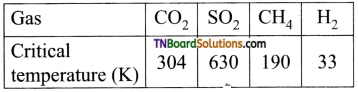(a) CO2
(b) SO2
(c) CH4
(d) H2
(d)
Hint: Higher the critical temperature, the greater is the adsorption. H2 has the least critical temperature and hence least adsorbed.

7. Which of the following are the characteristics of chemisorption?
(ii) Irreversibility
(iii) Low activation energy
(a) (i) and (ii) only
(b) (i) and (iii) only
(c) (ii) and (iii) only
(d) (i), (ii) and (iii)
(a)

8. The plot of $$\frac{x}{m}$$ (along Y-axis) versus log C (along X-axis) in the Freundlich adsorption isotherm is a horizontal line parallel to X-axis when,
(a) n = 0
(b) n = 1
(c) n = ∞
(d) such a plot is impossible
(c)
Hint: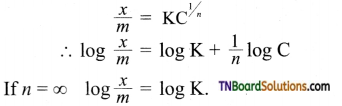i.e., constant parallel to log C axis.
[Note: This is the equation for adsorption of solids in solution.]

9. Match the following:

 A Fog 1 Gel B Milk 2 Foam C Cheese 3 Emulsion D Soap lather 4 Aerosol

Which of the following is the correct option?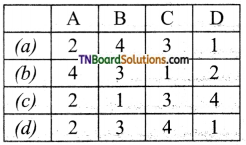(b)10. Which of the following will show the Tyndall effect?
(a) Aqueous solution of soap below critical miscelle concentration.
(b) Aqueous solution of soap above critical miscelle concentration.
(c) Aqueous solution of sodium chloride
(d) Aqueous solution of sugar
(b)

11. Method by which lyophobic sol can be protected:
(a) addition of oppositely charged sol
(d) by boiling
(c)

12. Freshly prepared precipitate sometimes gets converted to colloidal solution by:
(a) coagulation
(b) electrolysis
(c) diffusion
(d) peptisation
(d)

13. Which of the following electrolytes will have maximum coagulating value for AgI / Ag+ Sol?
(a) Na2S
(b) Na3PO4
(c) Na2SO4
(d) NaCl
(b)
Hint: AgI / Ag+ is a positively charged sol. Electrolytes whose negative ion (anion) has the least negative charge will require the maximum amount and will have the maximum coagulating value. Hence, Cl in NaCl.14. A colloidal system having a solid substance as a dispersed phase and liquid as dispersion medium is classified as:
(a) solid sol
(b) gel
(c) emulsion
(d) sol
(d)

15. Which of the following process is responsible for the formation of the delta at places where the river meets the sea?
(a) Emulsification
(b) Colloid formation
(c) Coagulation
(d) Peptisation
(c)
Hint: Formation of the delta-shaped heap of sand clay etc where the river falls into the sea due to coagulation of sand/clay by NaCl present in seawater.

16. When an excess of a very dilute aqueous solution of KI is added to a very dilute aqueous solution of silver nitrate, the colloidal particles of silver iodide are associated with which of the following Helmholtz double layer?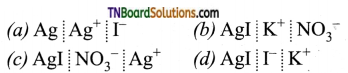(d)
Hint:
As excess KI has been added, I ions are adsorbed on AgI forming a fixed layer (giving negative charge). It then attracts the counter ion (K+) from the medium forming a second layer, (diffused layer).17. The ratio of a number of moles of AgNO3, Pb(NO3)2 and Fe(NO3)3 required for coagulation of a definite amount of colloidal sol of silver iodide prepared by mixing AgNO3 with an excess of KI will be:
(a) 1:2:3
(b) 3:2:1
(c) 6:3:2
(d) 2:3:6
(c)
Hint:
With excess KI, colloidal particles will be [AgI]I.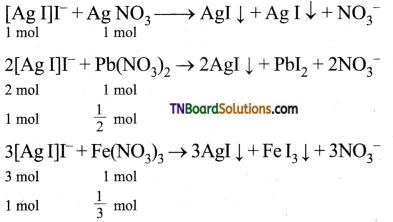Molar ratio required for coagulation of same amount of [AgI]I is 1 : $$\frac{1}{2}$$ : $$\frac{1}{3}$$ = 6 : 3 : 2.

18. Gelatin is mostly used in making ice creams in order to:
(a) prevent the formation of colloidal sol.
(b) enrich fragrance.
(c) prevent crystallisation and stabilise the mix.
(d) modify the taste.
(c)
Hint: Gelatin is a protective colloid. It stabilises the mix and prevents crystallisation.

19. Which of the following has a minimum gold number?
(a) Starch
(b) Sodium oleate
(c) Gum arabic
(d) Gelatin